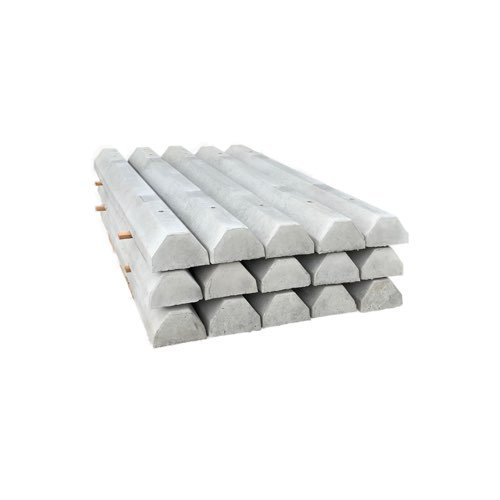# Supplementary Angles

The angles which make the summation of 180 degrees are known as supplementary angles. For example – Angles 130 degrees and 50 degrees are supplementary angles as the total or the summation of these angles will be equal to 180 degrees. While complementary angles are those angles that make 90 degrees. Suppose when we align the adjacent supplementary angles it will unite and form a straight line as well as a straight angle. Also, this should be focused on how the two complementary angles are not always next to one another. Thus any two angles that add up to 180 degrees form the supplementary angles.

## Properties of Supplementary AnglesSome of the important properties of the supplementary angles are as follows:

• The two angles are known as supplementary angles when they add up to make a 180°.
• The two angles together will make a straight line, while the angles are not required to be together.
• The “S” in the supplementary angles stands for the word “Straight” line. This means that they form 180 degrees.

## Examples Some examples of supplementary angles are as follows:

• 120° + 60° = 180°
• 90° + 90° = 180°
• 140° + 40° = 180°
• 96° + 84° = 180°

## How is Geometry Concerned Here?

As known, Geometry is a subject which is concerned with the study of all its various forms. Geometry is the first subject where we learn about the lines, angles of different structures. A straight system can be described as the distance between two locations without any bends or turns in between them. When the line segment joins at a single point it then forms an angle.

## Complementary Angles

The complementary angles are those angles in which the summation of two angles is equal to 90 degrees. In other words, if the two complementary angles add up it will form a right angle – when this happens they are referred to as the complementary angles. Also, we can say that these two angles complement each other. In another way, we can say that –

• sin (90°- A) = cos A and cos (90°- A) = sin A
• tan (90°- A) = cot A and cot (90°- A) = tan A
• sec (90°- A) = cosec A and cosec (90°- A) = sec A

We see from the above trigonometric equation that the ratio of the angles gets altered if they complement each other accordingly.

## Difference Between Complementary and Supplementary Angles

The difference between the complementary and Supplementary angles are as follows:

 Complementary Angles Supplementary Angles The summation of the complementary angles is equal to 90 degrees. The sum of the supplementary angles is equal to 180 degrees. The two angles will complement each other. The two angles are said to supplement each other They are not defined to be the linear pair of angles. These are defined to be the linear pair of angles. These angles are meant only for right angles. These angles are only for straight lines.

## Know the Trick!

How can you easily remember the difference between the Complementary angle and the supplementary angles? Very Simple!

1. Complementary angle starts with the letter ‘C’ and this ‘C’ stands for ‘Corner’ as well. The Corner makes it a 90 degree and thus they are at the right angle.
2. Supplementary angle starts with the letter ‘S’ and thus they stand for ‘Straight’ as in the straight line making it 180 degrees.